Next: Kepler's third law Up: Keplerian orbits Previous: Kepler's second law

# Kepler's first law

Our planet's radial equation of motion, Equation (4.18), can be combined with Equation (4.22) to give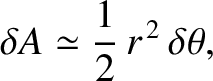(4.25)

Suppose that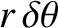, where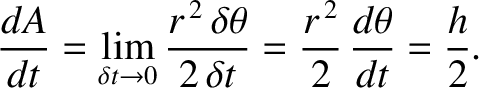and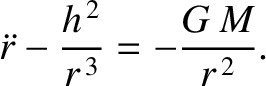. It follows that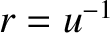(4.26)

Likewise,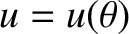(4.27)

Hence, Equation (4.25) can be written in the linear form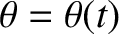(4.28)

As can be seen via inspection, the general solution to the preceding equation is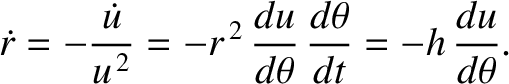(4.29)

where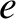and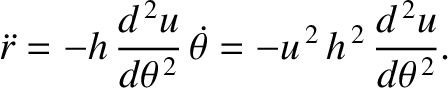are arbitrary constants. Without loss of generality, we can set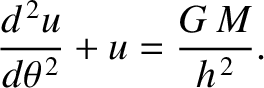by rotating our coordinate system about the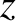-axis. We can also assume that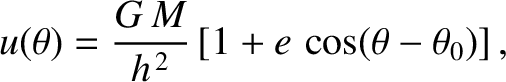. Thus, we obtain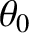(4.30)

where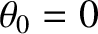(4.31)

Equation (4.30) is the equation of a conic section that is confocal with the origin (i.e., with the Sun). (See Section A.9.) Specifically, for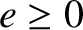, Equation (4.30) is the equation of an ellipse; for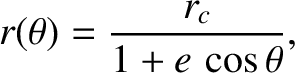, Equation (4.30) is the equation of a parabola; finally, for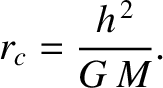, Equation (4.30) is the equation of a hyperbola. However, a planet cannot have a parabolic or a hyperbolic orbit, because such orbits are only appropriate to objects that are ultimately able to escape from the Sun's gravitational field. Thus, the orbit of our planet is an ellipse that is confocal with the Sun--this is Kepler's first law of planetary motionNext: Kepler's third law Up: Keplerian orbits Previous: Kepler's second law
Richard Fitzpatrick 2016-03-31